Next: Steady Temperature in a Up: EXAMPLES, TEMPERATURE FROM GF Previous: Solid cylinder with internal

## Solid sphere with convection.

Find the temperature in a solid sphere of radius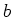, initially at elevated temperature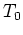, that is suddenly immersed in a fluid at constant temperature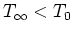. The heat transfer coefficient for the process is a constant value,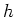. The temperature satisfies the following equations: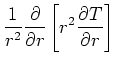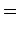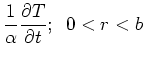(26)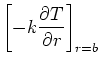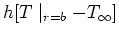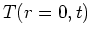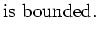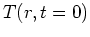As stated, this is case RS03B1T1. Note that the convection boundary condition provides that the heat flux at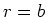will be positive for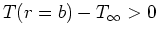. There are two driving terms, however one of them can be made homogeneous by suitable choice of a normalized temperature. (Generally it is better to zero out boundary conditions in favor of initial conditions but the purpose of this example is to demonstrate the convection boundary term.) Let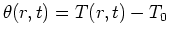. Then the differential equation is unchanged, the initial condition is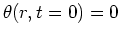, and the boundary condition may be written in standard form as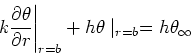Where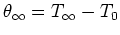. The temperature is given by the boundary-heating term of the GF solution equation in the form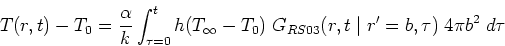(27)

The large-time GF for this case is given by: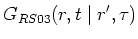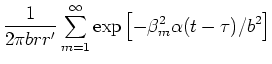(28)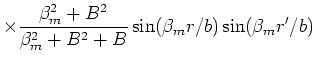where the eigenvalues are found from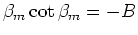and where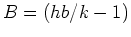. The time integral is easily evaluated to give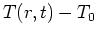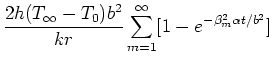(29)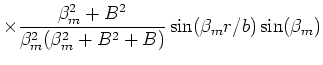For numerical evaluation the steady-state term of the series should be replaced by its constant value. As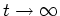, the sphere takes on the fluid temperature. That is,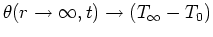. Substitute this constant value in place of the steady-state portion of the above series to find the following result: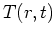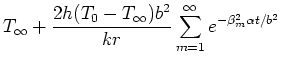(30)Note the sign change.Next: Steady Temperature in a Up: EXAMPLES, TEMPERATURE FROM GF Previous: Solid cylinder with internal
2004-01-31